# Lewis structure of acetone CH3COCH3

## What is the Lewis structure of acetone CH3COCH3?

Acetone has molecular formula CH3COCH3 and zero formal charge.

• First step: Choose the central atom. In this case, the carbonyl carbon atom (blue). Place all the other atoms around it by adding the valence electrons with the symbols “x” and “o”. Making sure that in contiguous atoms we have different types so as not to get confused.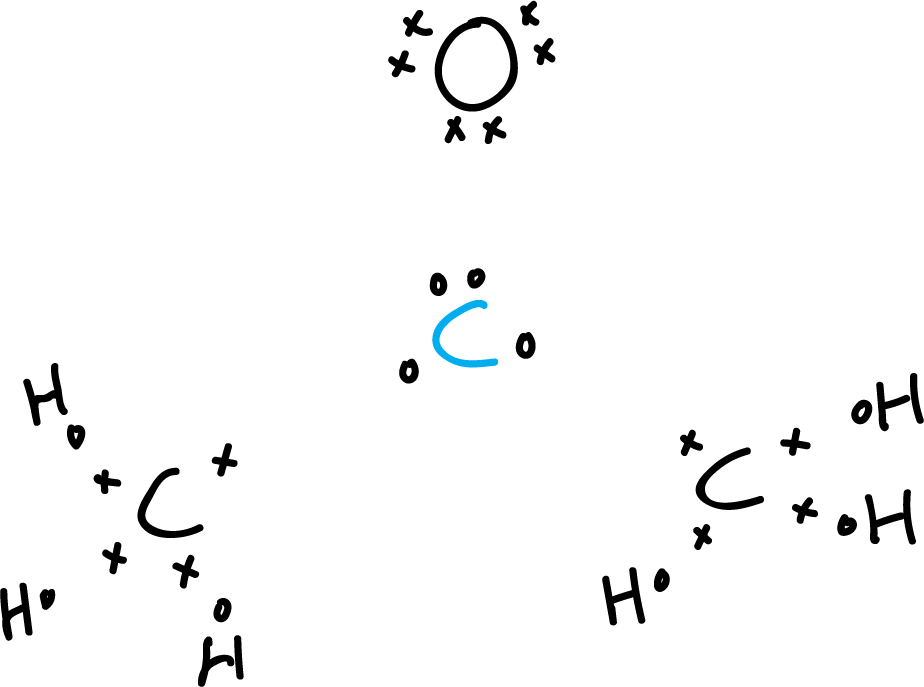• Second step: Count the valence electrons. The carbons present four valence electrons and
the oxygen presents 6, and the six hydrogens 1, each respectively.
This makes a total of (3 × 4) + (6 × 1) + (1 × 6)= 24.
Acetone has no net, extra or minus charge due to negative or positive ions.
Therefore, the total number of electrons is 24. We draw the resulting valence bonds (orange lines).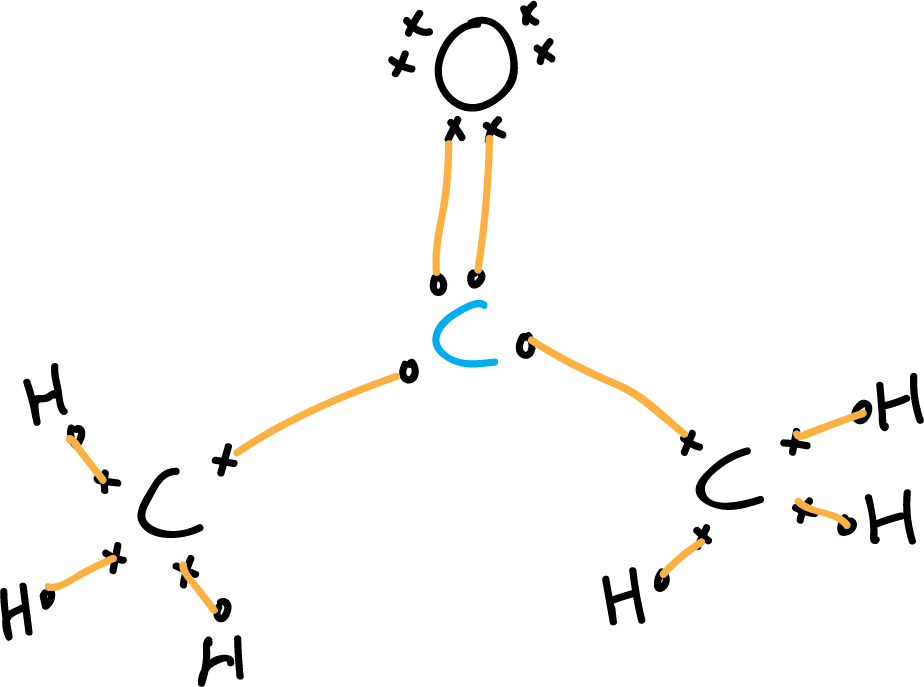• Third step: Locate the electron pairs and adjust to the octet rule.
The carbons are bonded to the central carbon (carbonyl carbon marked in blue).
We do the same with the nitrogen and with the hydrogens.
We look for the electrons needed to fulfill the octet rule.
As the four atoms (three carbons and one oxygen)
must fulfill it, the total number would be 4 x 8 = 32 electrons to fulfill the octet rule,
but we must subtract the 6 electrons of the hydrogens (32 – 6 = 26), so we are left with 26 electrons.
According to the previous calculations the number of bonding electrons
would be 26 – 24 = 1 corresponding to one more pair of the double bond of the carbonyl group. We draw the unbonded electron pairs (green lines).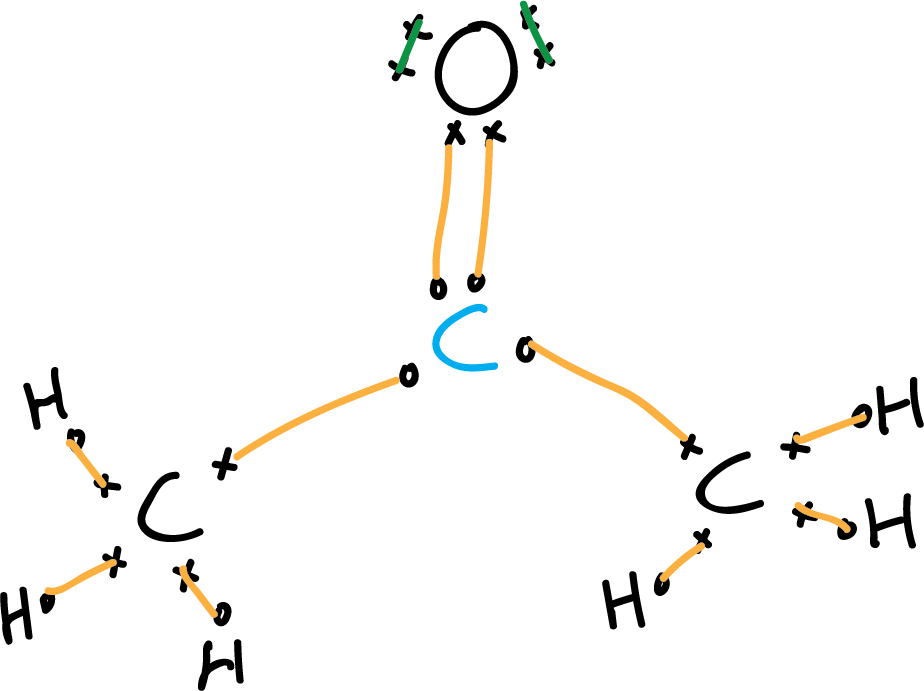• Fourth step: Non-bonding electrons (unshared electron pairs).
To locate the non-bonding electrons simply calculate the difference between the total valence electrons minus the bonding electrons: 32 – (26 + 2) = 4 electrons corresponding to 2 electron pairs, which are located under the premise of compliance with the octet rule are assigned to oxygen. (The drawing can be simplified by eliminating the electrons).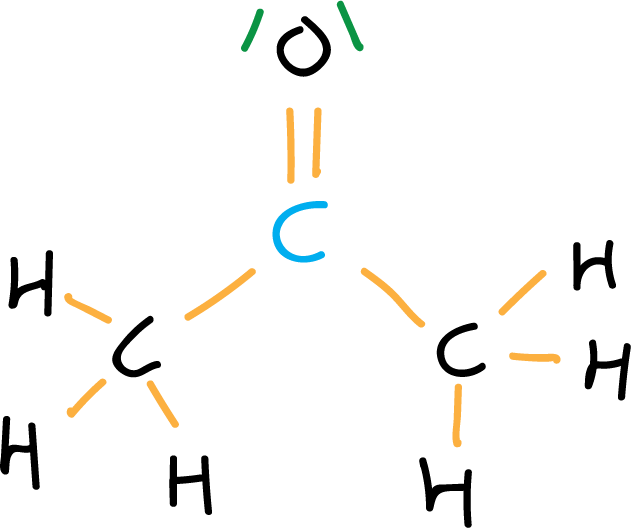• Fifth step: Formal charge. if formal charge exists, it is located on the corresponding atom. In this case the formal charge is zero. Generally, the representation of the acetone molecule is further simplified by eliminating the C-H bonds, and representing the electron pairs by dots.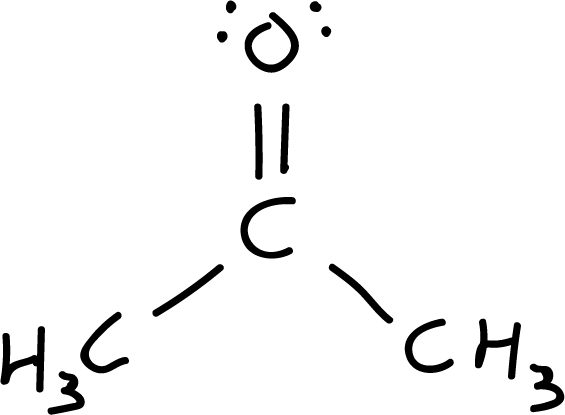• Step 6: Resonant structures. Resonant structures are explored by relocating charges and bonds. Starting from structure I, a pair of electrons is moved from the carbonyl double bond to the oxygen (more electronegative atom), resulting in the resonant structure II, which has a positive charge on the carbon and a negative charge on the oxygen (net charge is zero).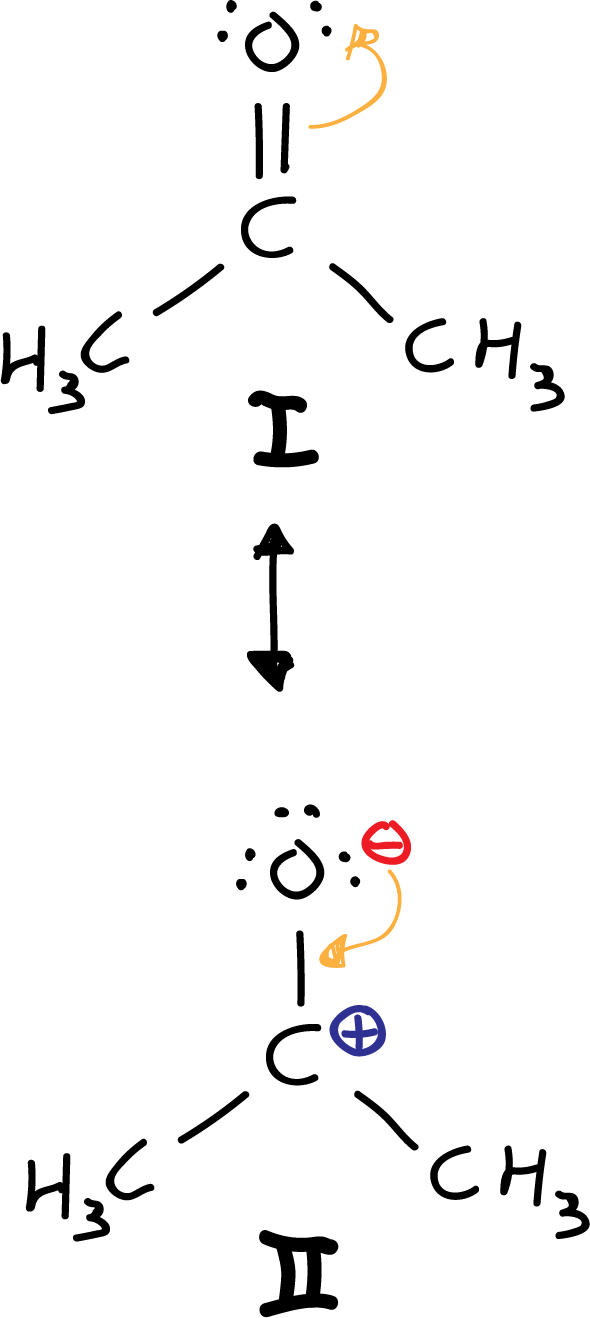Another option would be to move, from the I structure, a pair of electrons from the carbonyl double bond to the oxygen. However, because carbon is less electronegative (see electronegativity table) than oxygen, the III resonant structure is ruled out, because it results with an electronegative carbon and an electropositive oxygen.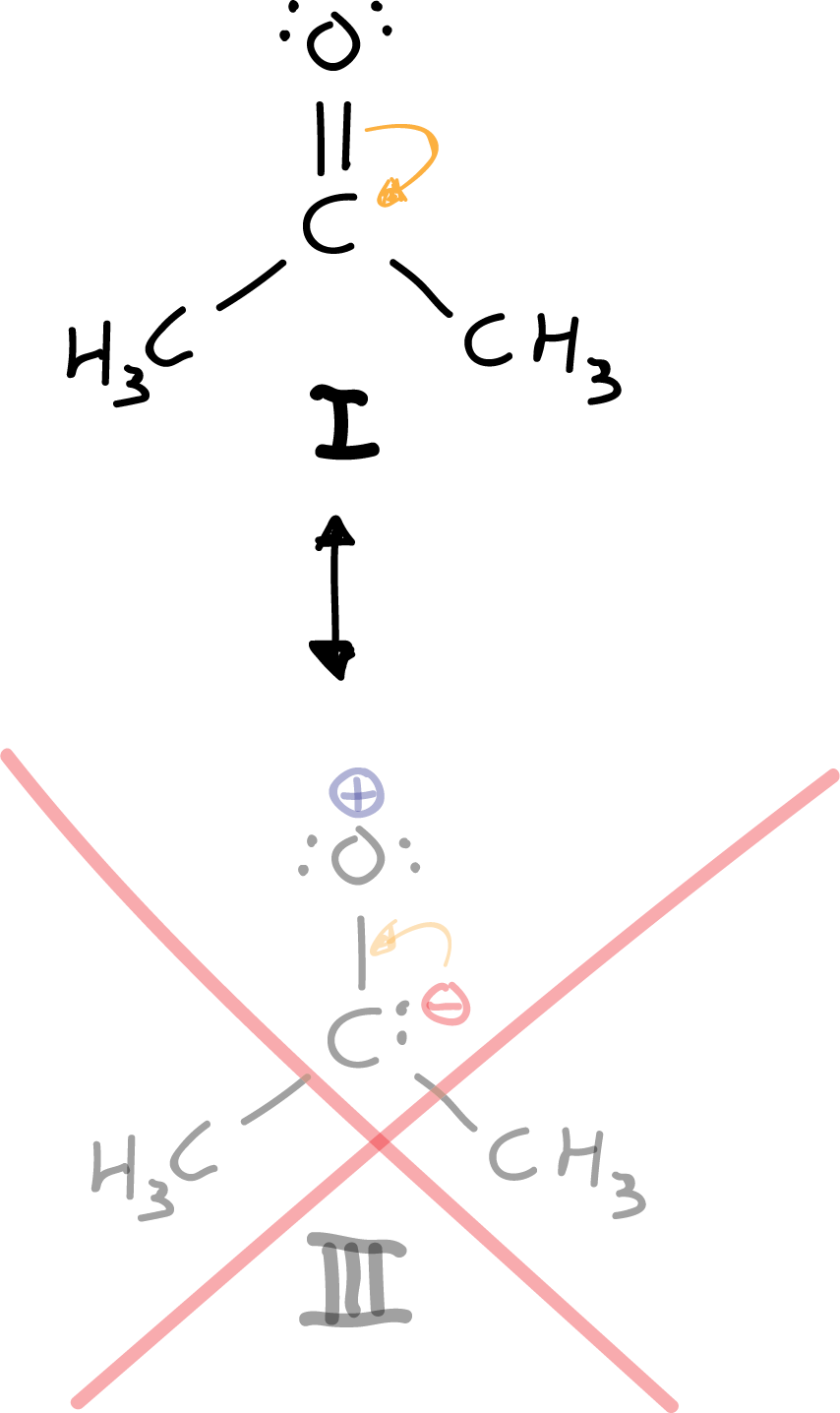There is only one resonant structure that complies with the octet rule, and that is structure I. Neither structure II nor IIIcomply with it, but III. In addition it is ruled out a priori because we are putting negative charge to an atom that is not the most electronegative of the molecule.

Shares